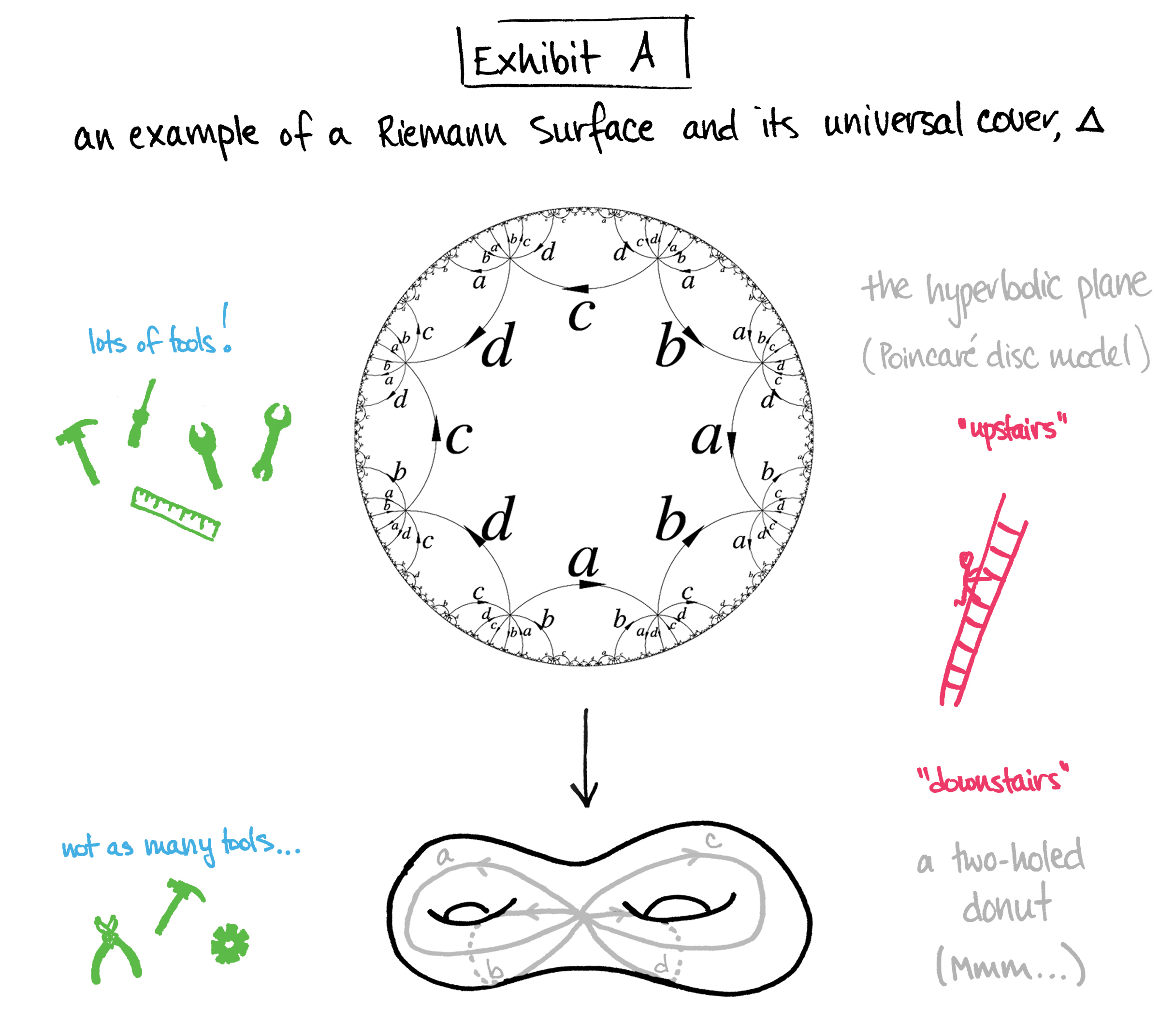# Three Important Riemann Surfaces

It occurred to me recently that my little blog is woefully short of posts about complex analysis. To remedy this situation, I've decided to use the next few posts to prove the following four facts:

But before I do, let's have

## A Little Motivation

Perhaps you're wondering,

Why should I care what the automorphisms of these four spaces look like? (Er, besides the fact that I need it for tonight's homework!)

Well, according to the uniformization theorem, every simply connected Riemann surface is conformally equivalent to either $\hat{\mathbb{C}}$, or $\mathbb{C}$, or $\Delta$ (and the latter is conformally equivalent to $\mathcal{U}$).

But why is this nice?

Because, from a topological viewpoint, it tells us that the universal cover of any Riemann surface will - up to conformal equivalence - either be $\hat{\mathbb{C}}$ or $\mathbb{C}$ or $\Delta$!

Okay, so?

Soooo, the universal cover is a very important object in mathematics. And the fact that there are  only three options (complex-analytically speaking) for the universal cover of any given Riemann surface is quite nice!

And why are universal covers so important?!

In short, it's because they make life easier! You see, sometimes it can be difficult to prove a result on a topological space $X$ (say, a Riemann surface). So to get around the difficulty, you “lift” the mathematics “upstairs” to the universal cover where - presumably - you have more tools at your disposal. (In our case, we have lots of tools at our disposal because we know TONS about $\mathbb{C}$ and hence $\hat{\mathbb{C}}$ and $\Delta$ as well.) Then after solving your problem upstairs, you can then project the result back down onto X. And VOILA! Result proved. Crisis averted.‍By placing a hyperbolic metric on the unit disc, we can use it to model the hyperbolic plane (aka the 'Poincare Disc Model'). In this light, the unit disc serves as the universal cover for our two-holed donut. (The image of the Poincare disc was taken from  here .)

This - the idea of lifting your (mathematical) problems to the universal cover - is just one technique that mathematicians use to gain information about a space $X$. Another well-known technique is to study functions on or to $X$. (For example, one may want to study functions $f$ from a circle $S^1$ into $X$. This results in a very powerful tool called the fundamental group.) In particular, one can study maps from $X$ to itself - and those are precisely the automorphisms of the space.

So you see? We really do care about the automorphisms of $\hat{\mathbb{C}}$, $\mathbb{C}$, and $\Delta\cong\mathcal{U}$! (To my more knowledgeable readers: feel free to provide us with more motivation in the comments.)

So far I've just rambled on a bit, but I hope this gives you at least a little motivation/background for the proofs in this series. Admittedly, the next few posts will be mostly computational and thus - in my opinion - not terribly exciting. But even so, I want to include them on the blog just in case a student or two may find them helpful.

Next time, I'll start by proving that all automorphisms of the unit disc $\Delta$ can be expressed in the form $f(z)=\frac{az+b}{\bar bz+\bar a}$ where $a,b\in\mathbb{C}$ satisfy $|a|^2-|b|^2=1$.

Until then!

Share
Related Posts